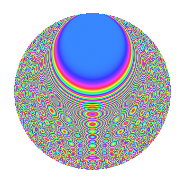# Properties

 Label 1050.2.bgLevel 1050 Weight 2 Character orbit bg Rep. character $$\chi_{1050}(121,\cdot)$$ Character field $$\Q(\zeta_{15})$$ Dimension 320 Sturm bound 480

# Related objects

## Defining parameters

 Level: $$N$$ = $$1050 = 2 \cdot 3 \cdot 5^{2} \cdot 7$$ Weight: $$k$$ = $$2$$ Character orbit: $$[\chi]$$ = 1050.bg (of order $$15$$ and degree $$8$$) Character conductor: $$\operatorname{cond}(\chi)$$ = $$175$$ Character field: $$\Q(\zeta_{15})$$ Sturm bound: $$480$$

## Dimensions

The following table gives the dimensions of various subspaces of $$M_{2}(1050, [\chi])$$.

Total New Old
Modular forms 1984 320 1664
Cusp forms 1856 320 1536
Eisenstein series 128 0 128

## Trace form

 $$320q + 40q^{4} + 4q^{5} + 8q^{6} - 12q^{7} + 40q^{9} + O(q^{10})$$ $$320q + 40q^{4} + 4q^{5} + 8q^{6} - 12q^{7} + 40q^{9} + 2q^{10} - 12q^{11} - 4q^{15} + 40q^{16} + 24q^{17} - 8q^{19} - 8q^{20} - 32q^{22} + 12q^{23} + 16q^{24} - 6q^{25} + 22q^{28} - 48q^{29} + 16q^{30} - 6q^{31} + 4q^{33} + 32q^{34} + 40q^{35} - 80q^{36} + 16q^{37} + 16q^{38} + 2q^{40} + 72q^{41} + 6q^{42} + 144q^{43} + 8q^{44} + 4q^{45} + 12q^{46} - 40q^{47} - 12q^{49} + 16q^{50} + 72q^{53} - 4q^{54} - 12q^{55} + 16q^{57} - 28q^{58} - 24q^{59} - 8q^{60} - 16q^{61} + 72q^{62} + 20q^{63} - 80q^{64} - 16q^{65} - 16q^{66} - 16q^{67} - 16q^{68} + 32q^{69} + 22q^{70} + 64q^{71} - 24q^{73} - 32q^{74} + 8q^{75} - 64q^{76} + 80q^{77} - 32q^{78} - 12q^{79} + 4q^{80} + 40q^{81} - 64q^{82} + 88q^{83} - 96q^{85} + 24q^{86} + 28q^{87} - 14q^{88} - 4q^{90} + 68q^{91} + 16q^{92} + 32q^{93} - 16q^{94} + 68q^{95} - 4q^{96} + 60q^{97} + 32q^{98} - 16q^{99} + O(q^{100})$$

## Decomposition of $$S_{2}^{\mathrm{new}}(1050, [\chi])$$ into newform subspaces

The newforms in this space have not yet been added to the LMFDB.

## Decomposition of $$S_{2}^{\mathrm{old}}(1050, [\chi])$$ into lower level spaces

$$S_{2}^{\mathrm{old}}(1050, [\chi]) \cong$$ $$S_{2}^{\mathrm{new}}(175, [\chi])$$$$^{\oplus 4}$$$$\oplus$$$$S_{2}^{\mathrm{new}}(350, [\chi])$$$$^{\oplus 2}$$$$\oplus$$$$S_{2}^{\mathrm{new}}(525, [\chi])$$$$^{\oplus 2}$$

## Hecke Characteristic Polynomials

There are no characteristic polynomials of Hecke operators in the database